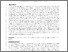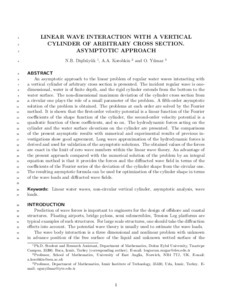# Linear Wave Interaction with a Vertical Cylinder of Arbitrary Cross Section: An Asymptotic Approach

Dişibüyük, N. B., and Yilmaz, O. (2017) Linear Wave Interaction with a Vertical Cylinder of Arbitrary Cross Section: An Asymptotic Approach. Journal of Waterway, Port, Coastal and Ocean Engineering, 143 (5). ISSN 0733-950XPreview PDF (Accepted manuscript) - Accepted Version Download (3MB) | Preview

## Abstract

An asymptotic approach to the linear problem of regular water waves interacting with a vertical cylinder of an arbitrary cross section is presented. The incident regular wave was one-dimensional, water was of finite depth, and the rigid cylinder extended from the bottom to the water surface. The nondimensional maximum deviation of the cylinder cross section from a circular one plays the role of a small parameter of the problem. A fifth-order asymptotic solution of the problem was obtained. The problems at each order were solved by the Fourier method. It is shown that the first-order velocity potential is a linear function of the Fourier coefficients of the shape function of the cylinder, the second-order velocity potential is a quadratic function of these coefficients, and so on. The hydrodynamic forces acting on the cylinder and the water surface elevations on the cylinder are presented. The present asymptotic results show good agreement with numerical and experimental results of previous investigations. Long-wave approximation of the hydrodynamic forces was derived and used for validation of the asymptotic solutions. The obtained values of the forces are exact in the limit of zero wave numbers within the linear wave theory. An advantage of the present approach compared with the numerical solution of the problem by an integral equation method is that it provides the forces and the diffracted wave field in terms of the coefficients of the Fourier series of the deviation of the cylinder shape from the circular one. The resulting asymptotic formula can be used for optimization of the cylinder shape in terms of the wave loads and diffracted wave fields.

Item Type: Article linear water waves,noncircular vertical cylinder,asymptotic analysis,wave loads Faculty of Science > School of Mathematics http://ascelibrary.org/doi/full/10.1061/... Pure Connector 23 Jun 2017 05:06 22 Oct 2022 02:47 https://ueaeprints.uea.ac.uk/id/eprint/63808 10.1061/(ASCE)WW.1943-5460.0000407View Item